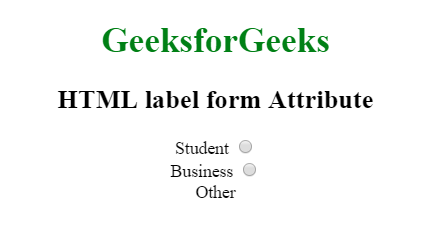# HTML | <label> form Attribute

The HTML <label> form Attribute is used to specify that the <label> element can contain one or more forms.

Syntax:

`<label form="form_id"> `

Attribute Values: It contains single value form_id which contains the value i.e form_id which specify one or more than the label element belongs to. The value of this attribute should be the id of the <form> element.

Example:

 `  ` `<``html``>  ` `    ``<``head``>  ` `        ``<``title``>  ` `            ``HTML label form Attribute  ` `        ``  ` `    ``  ` `     `  `    ``<``body` `style` `= ``"text-align:center"``>  ` `         `  `        ``<``h1` `style` `= ``"color:green;"``>  ` `            ``GeeksforGeeks  ` `        ``  ` `         `  `        ``<``h2``>  ` `            ``HTML label form Attribute  ` `        ``  ` `         `  `        ``<``form` `action``=``"#"` `method``=``"get"` `id``=``"GFG_form"``> ` `            ``` `            ``<``label` `for` `= ``"student"``>  ` `                ``Student  ` `            ``  ` `             `  `            ``<``input` `type` `= ``"radio"` `name` `= ``"Occupation"` `            ``id` `= ``"student"` `value` `= ``"student"``><``br``>  ` `     `  `            ``<``label` `for` `= ``"business"``>  ` `                ``Business  ` `            ``  ` `             `  `            ``<``input` `type` `= ``"radio"` `name` `= ``"Occupation"` `            ``id` `= ``"business"` `value` `= ``"business"``><``br``>  ` `        `` ` `         `  `        ``<``label` `for``=``"other"` `form``=``"GFG_form"``>Other ` `    ``  ` `     `  `                     `

Output:Supported Browsers: The browser supported by <label> form Attribute are listed below: